V1

2022/09/18阅读：117主题：凝夜紫

# 有向图的强连通分量问题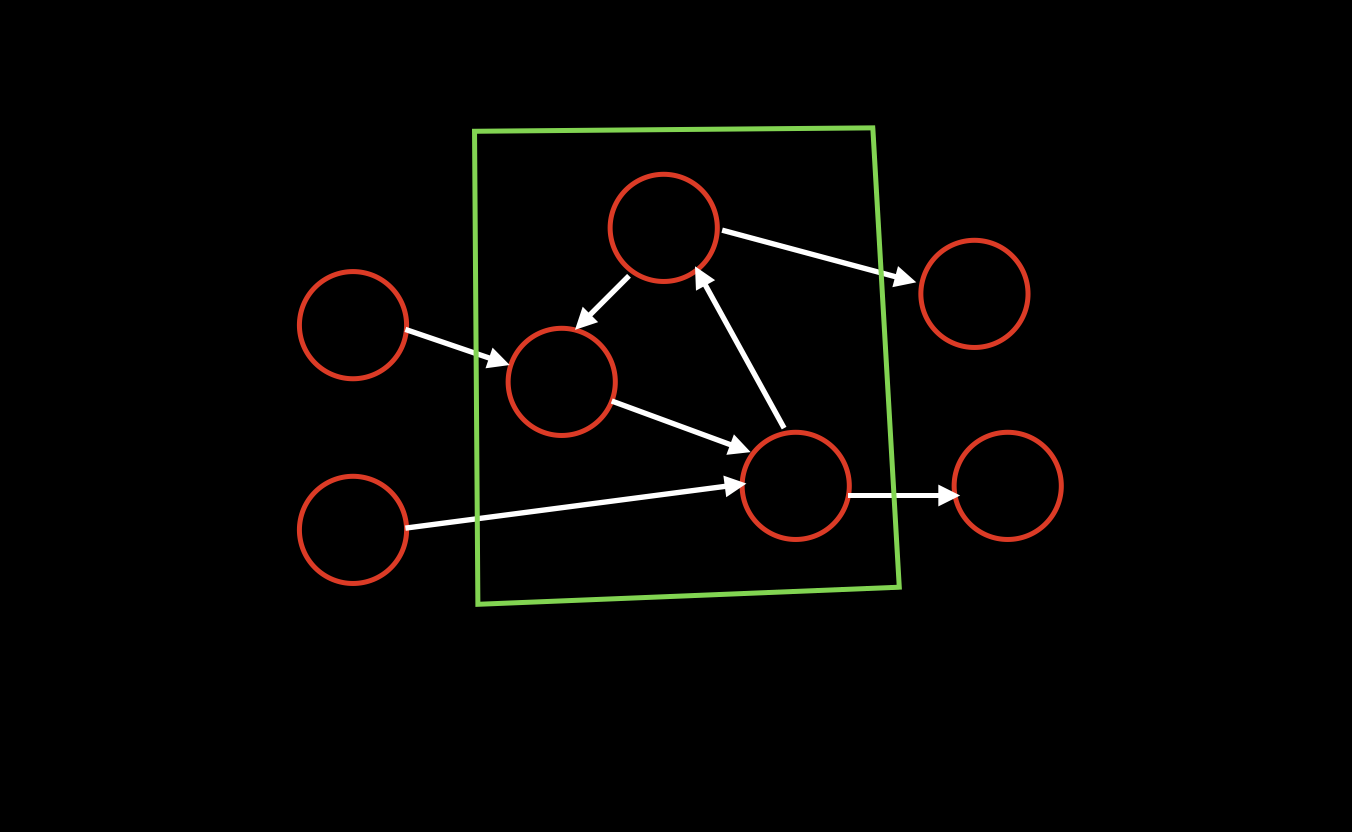在上图中，绿色部分就是强连通分量，所以我们可以把绿色部分缩点为一个点，同时其他四个单独的点，因为不和其他点相连，所以也符合强连通分量的定义，因此缩点后，整个图变成如下拓扑图的形式。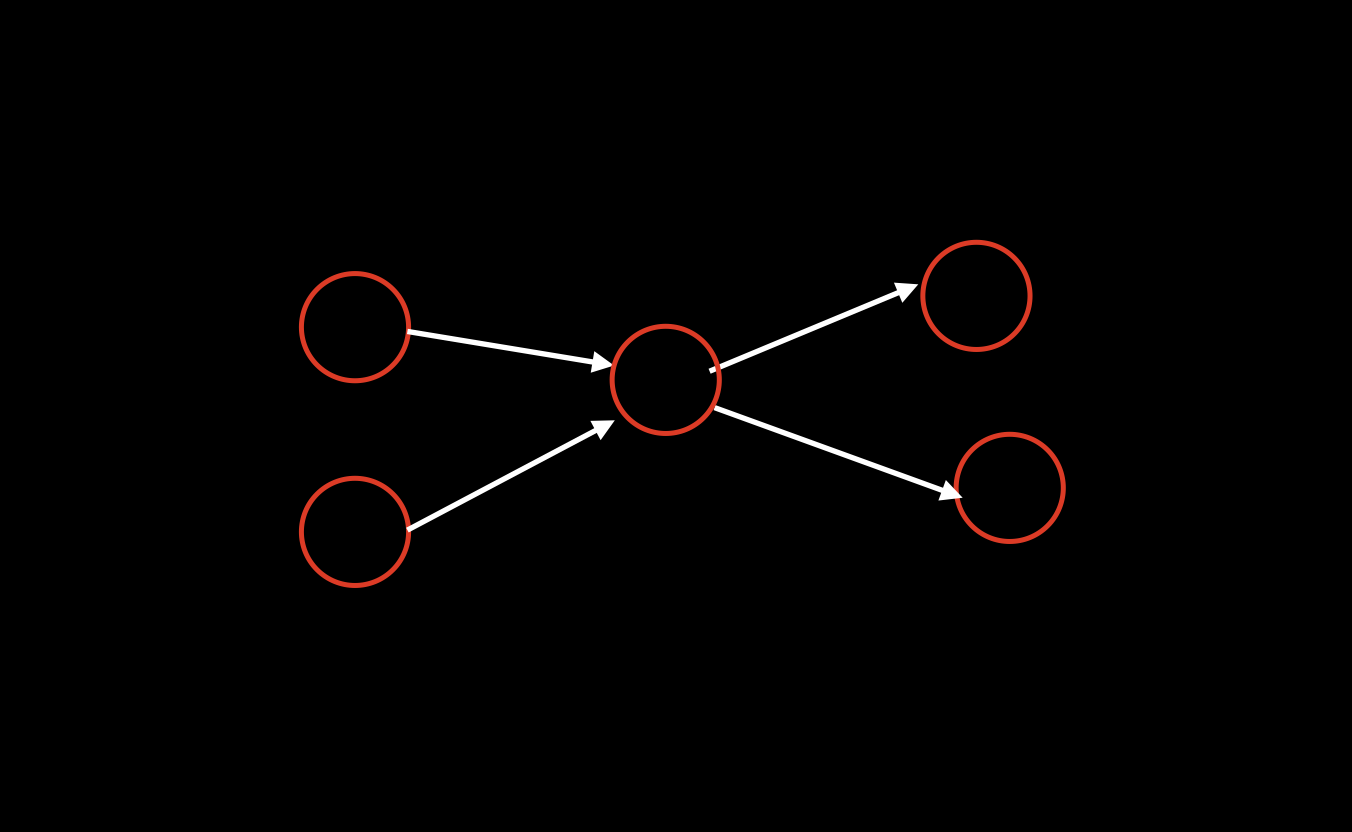## Tarjan算法求强连通分量

（ps: 关于这个部分，个人感觉真的是好难好难，可能也是之前没学过的原因，理解起来特别困难，在网上找了看了很多相关文章，大部分讲的都很好，但是并不适合初学者，希望我写的这个即便是初学者也能看完就懂。）

### 时间戳

1. `dfn[u]`：表示遍历到u时候的时间戳
2. `low[u]`：表示从u开始走，所能遍历到的最小的时间戳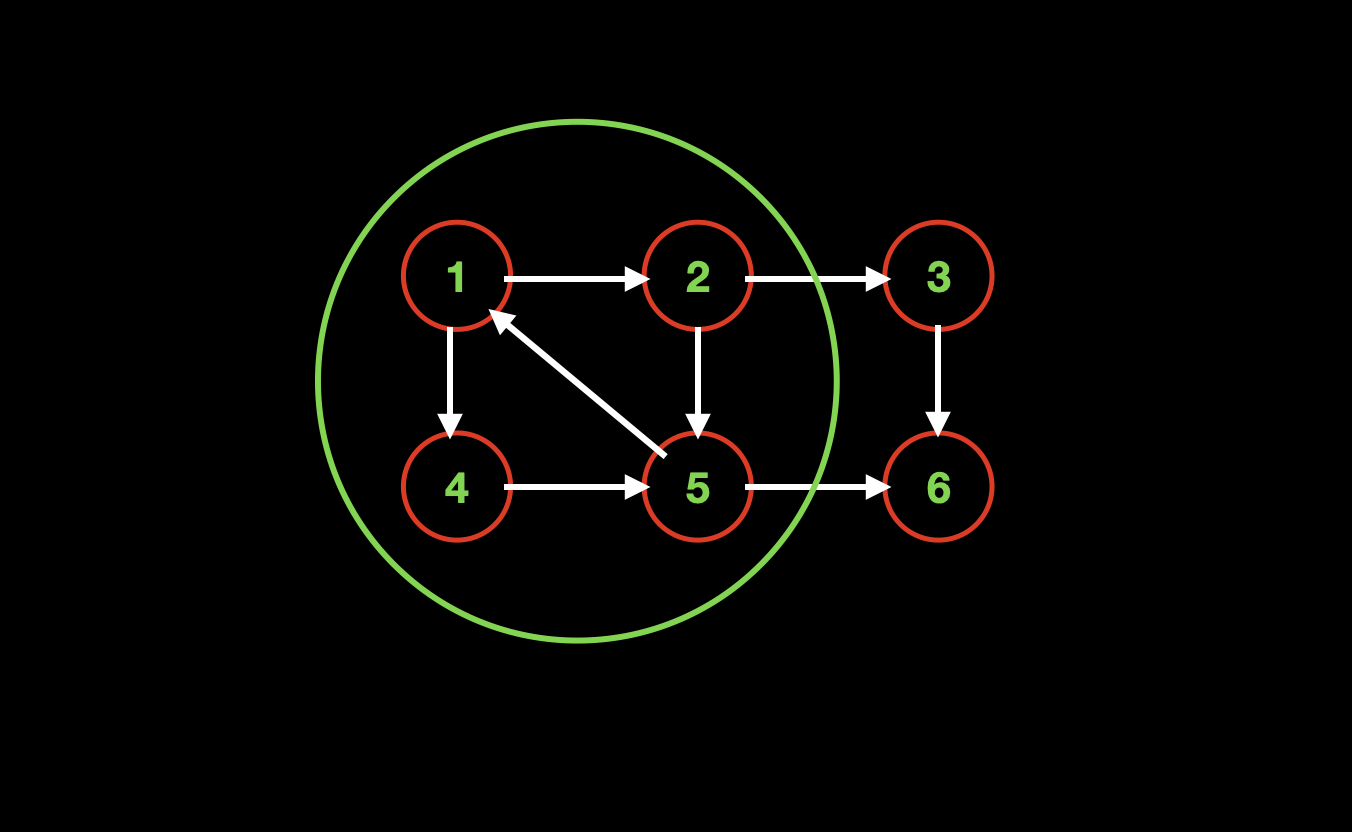这里，想用这个图来带大家直观的感受一下low[u]数组的含义
1. `u=1`的时候，`low[u]`是多少？根据low[u]数组的定义，毫无疑问是他自己，也就是`low=1`
2. `u=2`的时候，`low[u]`是多少？我们观察到图中绿色区域，其实是一个强连通分量，所以，根据强连通分量的定义（强连通分量中任意两点都是可以互相抵达的），我们知道`2`可以和`1`连通，那么根据`low[u]数组`的定义（low 所能遍历到的最小时间戳就是 1），也就是`low=1`;
3. 同理，因为`4``5`都在绿色的强连通分量中，所以他们也都能到达`1`，所以有`low=1``low=1`
4. 而对于`u=3`来说，只有两个点可以到达，一个是他自己，一个是`6`，所以很明显，从`3`开始走，能到达的最小点就是他自己，所以`low=3`;
5. 而当`u=6`，它就只能到达它自己了，所以`low=6`;

### 模板Code

``void dfs(int u) {    dfs[u] = ++ timestamp; // 给当前节点一个编号    // 遍历当前节点u的所有子节点    for (int i = h[u]; ~i; i = ne[i]) {        int j = e[i];        dfs(j); // 递归搜索所有子节点    }    // 当前分支搜索到底，也就是程序运行到这里的时候，    // 当前的u就是叶子节点，如果没有其他处理，    // 程序会因为所有语句执行完成而隐性的回溯，返回到上一个dfs(u)}``

``// tarjan求sccvoid tarjan(int u) {    dfn[u] = low[u] = ++ timestamp; // 代码1    stk[ ++ top] = u, in_stk[u] = true;  // 代码2    // 遍历出u的所有子节点j    for (int i = h[u]; ~i; i = ne[i]) {        int j = e[i];        if (dfn[j]) {  // 代码3            tarjan(j); // DFS序的遍历            low[u] = min(low[u], low[j]);  // 代码4        } else if (in_stk[j]) {            low[u] = min(low[u], dfn[j]); // 代码5        }    }        if (dfn[u] == low[u]) { // 代码6        int y;        ++ scc_cnt; // 给 强连通分量 设置一个编号        do {  // 代码7开始            y = stk[top -- ];              in_stk[y] = false;            id[y] = scc_cnt;        } while {y != u};  // 代码7结束    }}``
1. 代码1：初始化dfn和low两个数组，这里如果不理解，请回看上面的定义；

2. 代码2：`stk[]`数组存储遍历到的节点，`in_stk[]`数组记录节点u是否在stk数组中。所以这句代码的作用就是初始化，将当前节点u放到结构中，并在另一个标记数组中标记该节点`u`目前正在中。

3. 代码3：假如子节点`j`没有被遍历过(没被遍历过就是dfn[j]=0)，则进入递归。

4. 代码4：更新low[u]的第一处代码，从这句开始，变得烧脑了。这里为啥要用low[子节点]去更新low[父节点]呢？

注意这句代码的位置，它是在递归之后的，也就是搜索完当前分支并回溯的时候，才开始执行的

设想，以上面的low[u]数组讲解中的强连通分量 3 和 6为例，当`u=3`的时候`j=6`，并且有`low=3`，这个`low=3`是怎么更新出来的呢？没错，就是这句代码4`low[u] = min(low[u], low[j]);`更新出来的。

而从意义来看，`low[u]`表示`u`所能到达的点中编号最小的点，而`j`本来就是`u`所能到达的点，所以`low[j]`是完全有可能更新`low[u]`的。

综上所述，代码4存在的原因，我们就找到了，因为DFS遍历是必须回溯的，所以可以在回溯的时候通过比较父节点和子节点的low数组，来更新父节点的low数组

5. 代码5：更新low[u]的第二处代码，我们首先要注意这句代码的进入时机，这句代码是在子节点已经被拥有编号并且仍然在stk中才会执行，注意子节点已经在`stk`里，说明这句代码也是需要在回溯阶段执行的。（至于子节点仍在stk中的意义是什么，请往后看。）

因为`j``u`的子节点，也就是说可以从`u`到达`j`，那么根据`low[u]`的定义，`dfn[j]`就可能成为`u`能到达的点中编号最小的点，所以要用`dfn[j]`更新`low[u]`是合理的。

6. 代码6：还记得我们的重要性质吗？当触发`代码6`的时候，说明我们找到了一个强连通分量的开始，也就是说后面的代码，一定是把整个强连通分量都找出来。

7. 代码7：整个do...while循环都在做一件事情，把整个强连通分量找出来

如果想找出一个范围区间（类比强连通分量），我们需要知道什么？从直觉上来看，我们需要知道区间的起始节点和终止节点，由这两个节点来确定一个区间。事实上的确如此，但是如果我们把区间加入到数据结构中就变得不需要两个节点了，我们只需要知道一个起始节点就可以了，因为我们可以从当前起始节点开始出栈，一直到下一个起始节点终止，这样所有出栈的元素，其实就是属于同一个区间，在这个过程中，我们只需要知道每个区间的起始节点，而不需要知道终止节点。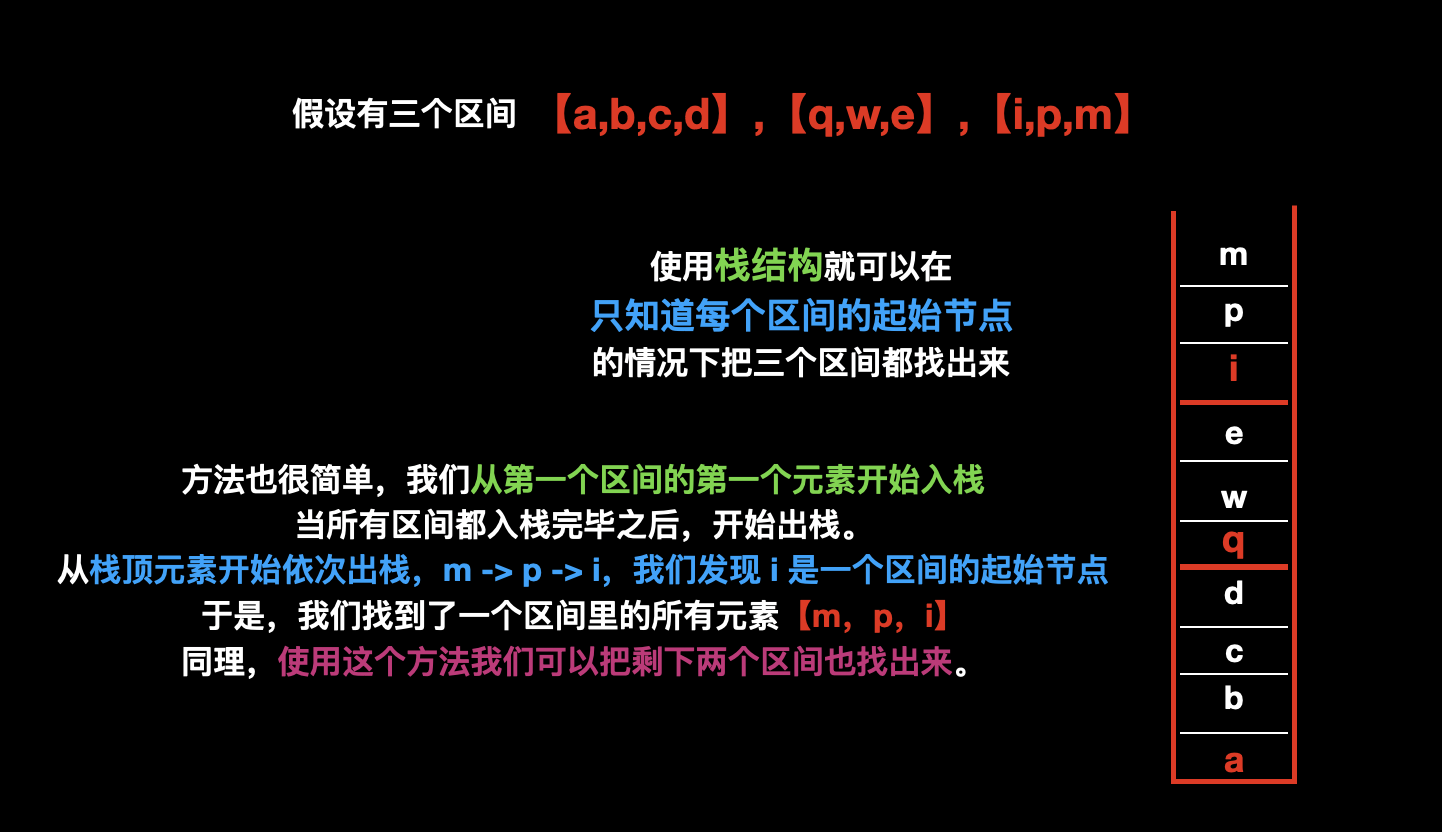明白了这个原理之后，读者再来看代码7这段代码片段，是否有种霍然开朗的感觉呢？

注意，代码7的执行时机是一个分支全部搜索结束的时候，做的事情实际上就是在栈顶弹出元素，直到弹出的元素是 起始节点（当前u），说明我们就找到了以当前u为最小根的强连通分量

## 缩点

### 模板Code

``// 遍历所有点for (int u = 1; u <= n; u ++ ) {    for (int i = h[u]; ~i; i = ne[i]) {        // j是u的子节点        int j = e[i];        // 如果u和j不在一个强连通分量        if (id[u] != id[j]) {               // 点u的出度 ++             dout[id[u]] ++ ;        }    }}``

# AcWing 1174. 受欢迎的牛

``````1≤N≤10^4,
1≤M≤5×10^4
``````

``````3 3
1 2
2 1
2 3
``````

``````1
``````

``````只有第三头牛被除自己之外的所有牛认为是受欢迎的。
``````

## 题目分析

1. 如果拓扑图存在两个点的出度为0，那么说明不存在牛满足目标。这点很好理解，因为这种情况，至少 出度为0的点 不会 被另一个出度为0的点 认为受欢迎。
2. 如果只有一个点出度为0，那么就表示其他所有点多能走到这个出度为0的点，也就是其他所有牛都认为它是受欢迎的。

## Code

``#include <iostream>#include <cstring>#include <algorithm>using namespace std;const int N = 10010, M = 50010;int h[N], e[M], ne[M], idx;int dfn[N], low[N], timestamp;int stk[N], top, id[N], scc_cnt;bool in_stk[N];int n, m, Size[N], dout[N];void add(int a, int b) {    e[idx] = b, ne[idx] = h[a], h[a] = idx ++ ;}void tarjan(int u) {    dfn[u] = low[u] = ++ timestamp;    stk[++ top] = u, in_stk[u] = true;    for (int i = h[u]; ~i; i = ne[i]) {        int j = e[i];        if (!dfn[j]) {            tarjan(j);            low[u] = min(low[j], low[u]);        } else if (in_stk[j]) {            low[u] = min(dfn[j], low[u]);        }    }    if (low[u] == dfn[u]) {        int y;        ++ scc_cnt;        do {            y = stk[top -- ];            in_stk[y] = false;            id[y] = scc_cnt;            // 记录每个强联通分量中点的数量            Size[scc_cnt] ++ ;        } while (y != u);    }}int main() {    cin >> n >> m;    memset(h, -1, sizeof h);    while (m -- ) {        int a, b;        scanf("%d%d", &a, &b);        add(a, b);    }    // tarjan 遍历出所有的强联通份量    for (int i = 1; i <= n; i ++ ) {        if (!dfn[i]) tarjan(i);    }    // 缩点    for (int u = 1; u <= n; u ++ ) {        for (int i = h[u]; ~i; i = ne[i]) {            int j = e[i];            int a = id[u], b = id[j];            // a的出度 +1             if (a != b) dout[a] ++ ;        }    }    int zeros = 0, sum = 0;    for (int i = 1; i <= scc_cnt; i ++ ) {        if (!dout[i]) {            zeros ++ ;            sum += Size[i];            if (zeros > 1) {                sum = 0;                break;            }        }    }    printf("%d\n", sum);    return 0;}``

# AcWing 367. 学校网络

``````2≤N≤100
``````

``````5
2 4 3 0
4 5 0
0
0
1 0
``````

``````1
2
``````

## 题目描述

1. 最少需要多少个软件，直接给学校，才能让网络中所有学校都获得；
2. 最少需要添加几个新的支援关系，才能让网络中，任意一个学校获得软件，其他所有学校也可以获得。

## 题目分析

### 问题1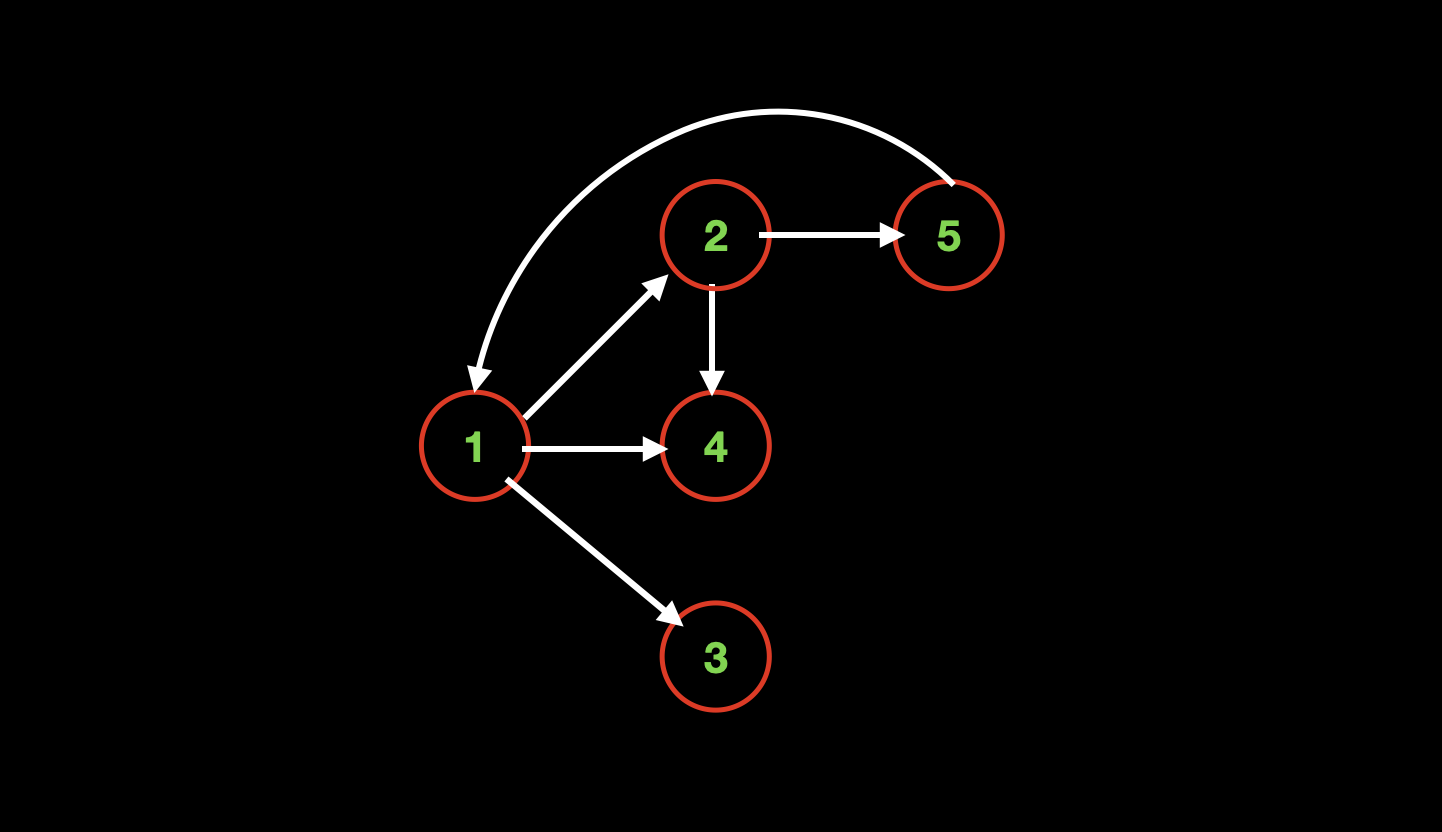根据题面中的给定示例，我们可以画出如上图。我们发现想让所有的学校都获得软件，只需要给`1`号点即可，因为1号点可以传给2,3,4，而2号点接到后，又会传给5号点，至此，所有的学校都获得了软件

### 问题2

1. `P==1`的时候，表示只有一个起始点，那么这个起点，由于这个图是有向无环图，所以该起点必然可以走到每个终点，此时，我们只需要把每一个终点向起点连一条边，就可以使整个图变成强连通分量。通过下图，我们可以直观的看到，当从每个终点连接一条边（绿色）到起点后，整个图变成了强连通分量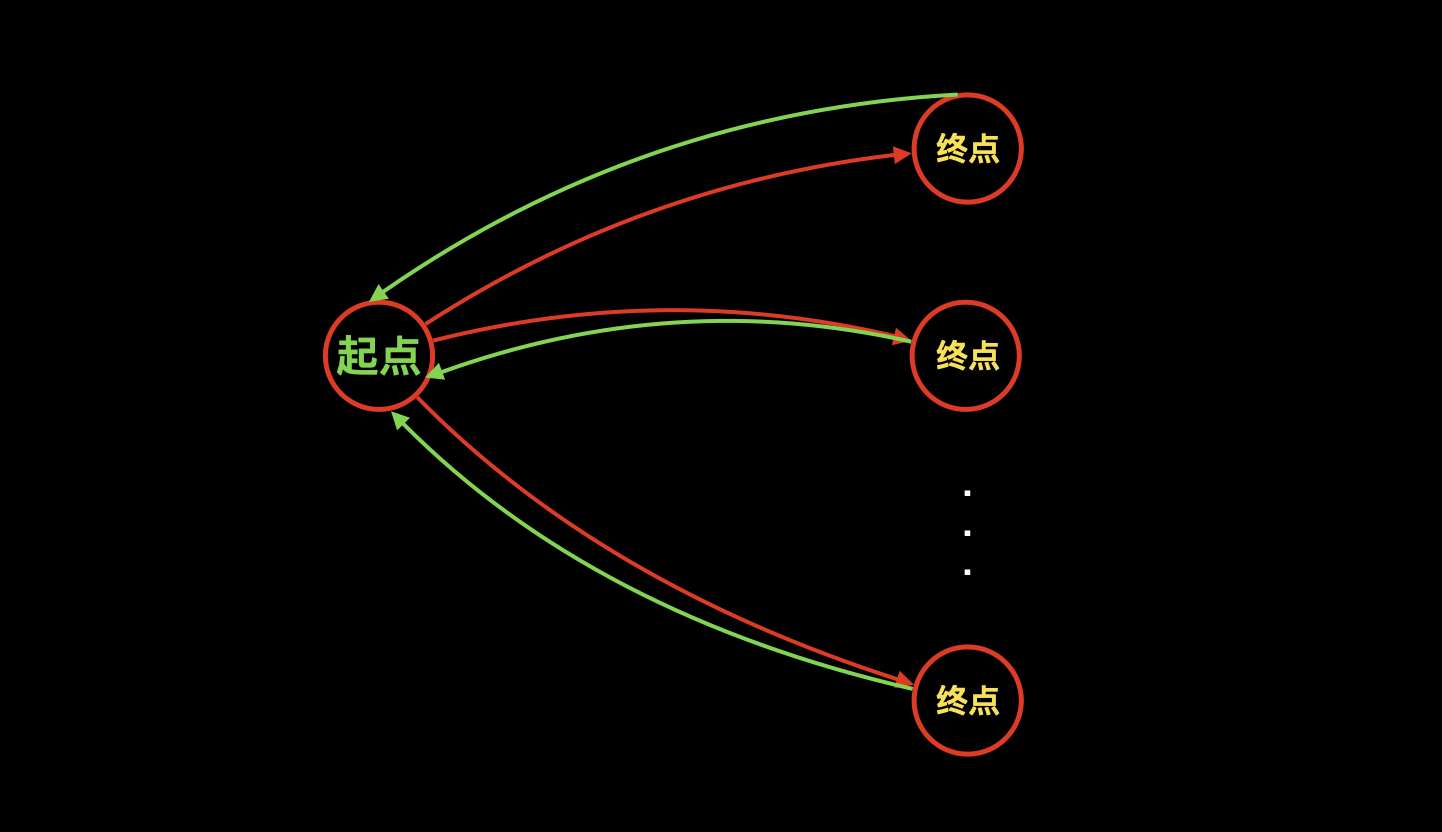2. `P>1`的时候，必然可以找到两组点满足

1. 某个起点P1可以走到某个终点Q1；
2. 某个起点P2可以走到某个终点Q2；

既，不同的起点，可以走到不同的终点

这一点是论证结论的核心，为什么一定会有不同的起点走到不同的终点呢？因为是`有向无环图`，这种图的每个非起点必须有前驱节点，假如好多起点都走到同一个终点Q1，那么说明Q2是孤立的点，与有向无环图定义不符。

这种情况下，我们把把某终点的边连接到某起点，这样子做会减少一个起点以及一个终点。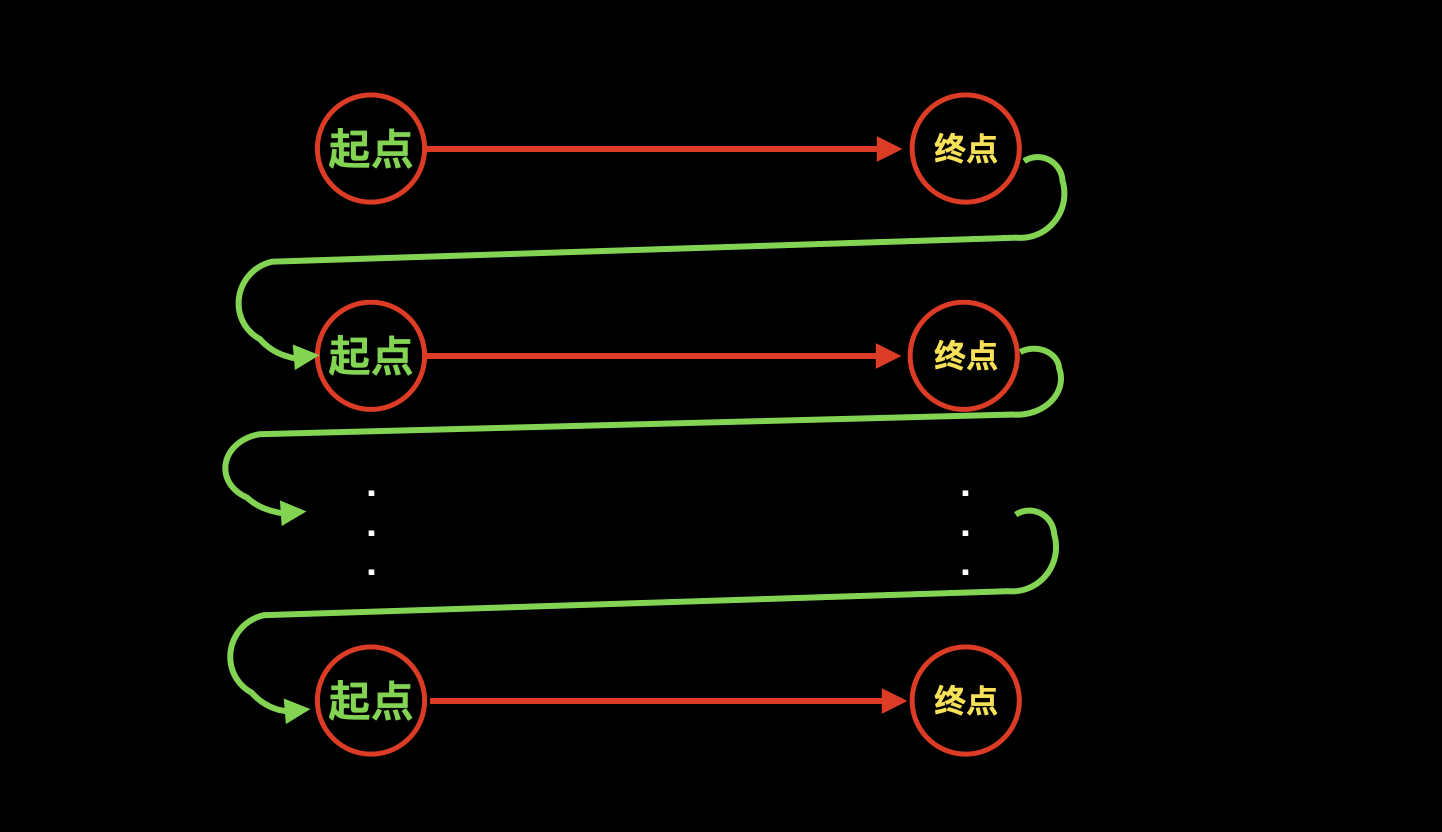原来有`P`个起点和`Q`个终点，每连接一条边都会使`P-=1``Q-=1`，那么我们只要一共连接`P-1`条边，`P`就会变成`1`，此时`Q`会变成`Q-(P-1)`

那么根据我们的大前提，Q是有可能大于P的。

此时，`P==1`,`Q>1`，还需要加多少条边呢？这一点我们在上一步`p==1`时候已经求出来了，既，Q是多少就需要加多少条边，那么此时`Q``Q-(P-1)`，所以也需要加这么多条边，再算上刚才增加的`P-1`条边，总共增加的边数量就是`Q-(P-1)+(P-1)=Q`

3. 以上原理，是在`P<=Q`的大前提下成立的，那么我们反过来`P>=Q`也是成立的，所以，就有最后的结论：`max(Q, P)`

## Code

``#include <iostream>#include <cstring>using namespace std;// 边的极限数量，每个点都是孤立的，也就需要N^2条边；const int  N = 110, M = N * N;int h[N], e[M], ne[M], idx;int dfn[N], low[N], timestamp;int stk[N], top;bool in_stk[N];int id[N], scc_cnt, din[N], dout[N];int n;void add(int a, int b) {    e[idx] = b, ne[idx] = h[a], h[a] = idx ++ ;}void tarjan(int u) {    dfn[u] = low[u] = ++ timestamp;    stk[++ top] = u, in_stk[u] = true;    for (int i = h[u]; ~i; i = ne[i]) {        int j = e[i];        if (!dfn[j]) {            tarjan(j);            low[u] = min(low[u], low[j]);        } else if (in_stk[j]) {            low[u] = min(low[u], dfn[j]);        }    }    if (dfn[u] == low[u]) {        ++ scc_cnt;        int y;        do {            y = stk[top -- ];            id[y] = scc_cnt;            in_stk[y] = false;        } while (y != u);    }}int main() {    cin >> n;    memset(h, -1, sizeof h);    for (int i = 1; i <= n; i ++ ) {        int a;        while (cin >> a, a) add(i, a);    }    // 求所有的 强连通分量    for (int i = 1; i <= n; i ++ )        if (!dfn[i]) tarjan(i);    // 缩点    for (int u = 1; u <= n; u ++ ) {        for (int i = h[u]; ~i; i = ne[i]) {            int j = e[i];            int a = id[u], b = id[j];            // a 向 b 连接  则a的出度 ++； b的入度 ++ ；            if (a != b) dout[a] ++ , din[b] ++ ;        }    }    int P = 0, Q = 0;    for (int i = 1; i <= scc_cnt; i ++ ) {        if (!din[i]) P ++ ; // 没有入度 说明是起点        if (!dout[i]) Q ++ ;  // 没有出度 说明是终点    }    printf("%d\n", P);  // 问题1 统计起点    //特判 如果整个图本来就是 一个强连通分量 则不需要加任何的边    if (scc_cnt == 1) puts("0");     else printf("%d", max(P, Q)); // 问题2    return 0;}``

# AcWing 1175. 最大半连通子图

``````1≤N≤10^5,
1≤M≤10^6,
1≤X≤10^8
``````

``````6 6 20070603
1 2
2 1
1 3
2 4
5 6
6 4
``````

``````3
3
``````

## 题目描述

1. 有向图半连通：强连通中，所有点两两之间，存在双向边，既，u指向v，v也必须指向u；而半连通中，所有点两两之间，存在单向边即可，u指向v 或者 v指向u 均可

2. 导出子图：从原图中选出一些点和一些边，如果选出的边都是与选出点相关的，说明选出的是导出子图。其中“相关”的含义是选出边的两个点，均是选出点。

3. 最大半连通子图：如果导出子图是半连通的，并且该导出子图，是原图所有导出子图中点数最多的

## 题目分析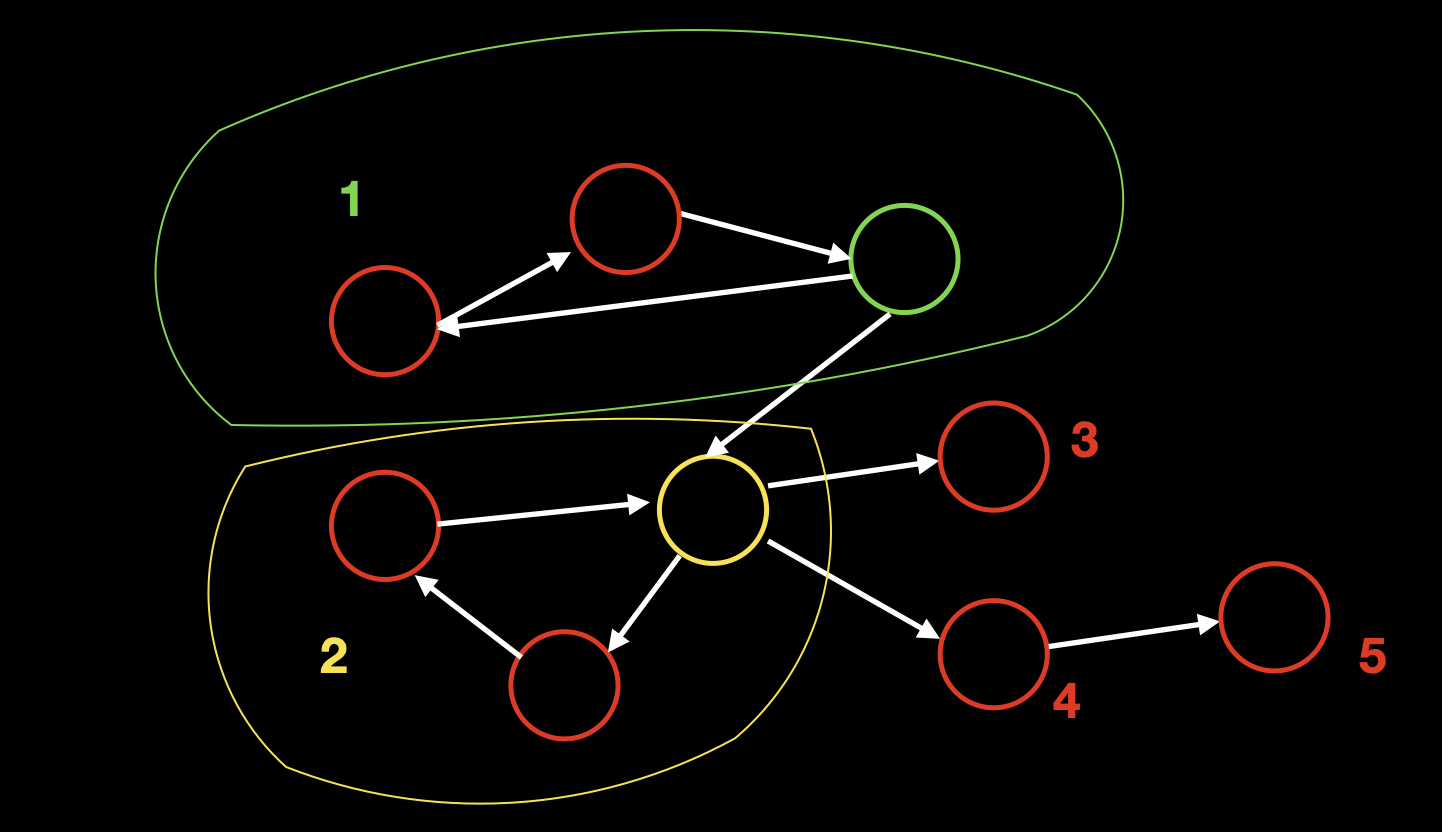图中共有5个强连通分量，其中1号区域是强连通分量，所以1号区域中所有点都能走到绿色点；同理，2号区域所有点都能走到黄色点。又因为绿色点也能走到黄色点，所以1号区域所有点可以走到2号区域所有点，满足半连通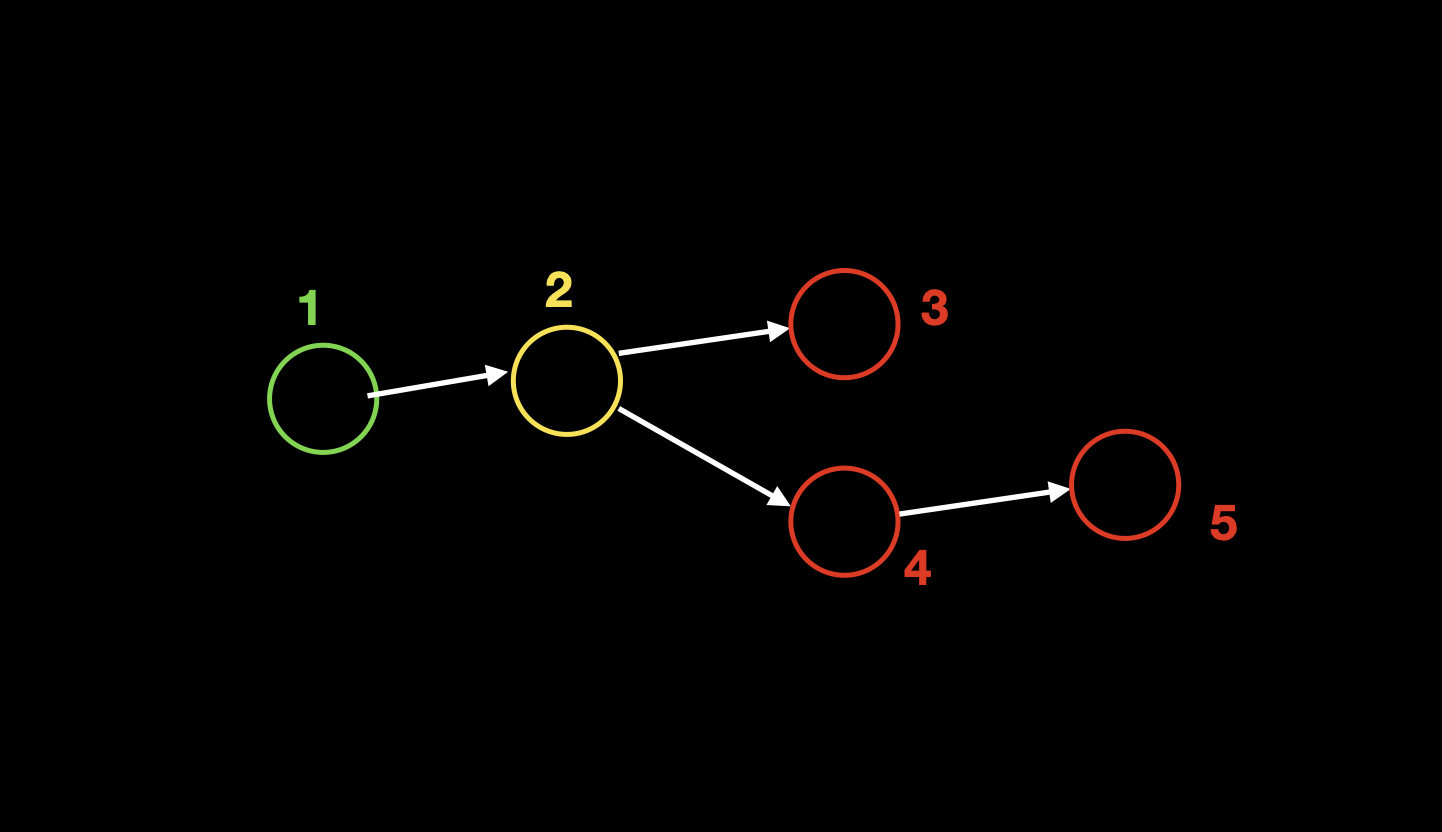缩点成有向无环图后，我们发现形成了两条分支，而属于不同分支的两个区域`3``5`是不可达的，不满足半连通。所以这个有向无环图中，存在两个半连通子图

1. 1->2->3;
2. 1->2->4->5;

## Code

1. f[i]表示以f[i]为结尾的点的最大数量，状态转移就是从i的所有前驱节点j中取f[j]的最大值。
2. g[i]表示以f[i]的路线数量。
``#include <iostream>#include <cstring>#include <unordered_set>using namespace std;typedef long long LL;const int N = 100010, M = 2000010;int h[N], hs[N], e[M], ne[M], idx;int dfn[N], low[N], timestamp, stk[N], top, scc_cnt;bool in_stk[N];int id[N], Size[N]; // Size表示每个强连通分量中点的数量int n, m, X;int f[N], g[N];void add(int h[], int a, int b) {    e[idx] = b, ne[idx] = h[a], h[a] = idx ++ ;}void tarjan(int u) {    dfn[u] = low[u] = ++ timestamp;    stk[++ top] = u, in_stk[u] = true;    for (int i = h[u]; ~i; i = ne[i]) {        int j = e[i];        if (!dfn[j]) {            tarjan(j);            low[u] = min(low[u], low[j]);        } else if (in_stk[j]) low[u] = min(low[u], dfn[j]);    }    if (dfn[u] == low[u]) {        int y;        ++ scc_cnt;        do {            y = stk[top -- ];            in_stk[y] = false;            id[y] = scc_cnt;            Size[scc_cnt] ++ ;        } while (y != u);    }}int main() {    memset(h, -1, sizeof h);    memset(hs, -1, sizeof hs);    cin >> n  >> m >> X;    while (m -- ) {        int a, b;        scanf("%d%d", &a, &b);        add(h, a, b);    }    for (int i = 1; i <= n; i ++ )        if (!dfn[i]) tarjan(i);    // 用来判断边是否重复 哈希函数 (v, u) -> v * 1000000 + u    unordered_set<LL> S;     for (int u = 1; u <= n; u ++ )        for (int i = h[u]; ~i; i = ne[i]) {            int j = e[i];            int a = id[u], b = id[j];            LL hash = a * 1000000ll + b; // 乘法可能爆int 转LL            if (a != b && !S.count(hash)) {                S.insert(hash);                add(hs, a, b);            }        }    // 遍历新图（缩点后的有向无环图） 计算    for (int u = scc_cnt; u; u -- ) {        // 未被更新过的f[u]就是起点 初始化起点        if (!f[u]) {            f[u] = Size[u];            g[u] = 1;        }        // 遍历 u能到达的点 j         for (int i = hs[u]; ~i; i = ne[i]) {            int j = e[i];            // 状态转移 f[j] 等于它的父节点的总点数f[u]+它自己的点数scc_cnt[j]            if (f[j] < f[u] + Size[j]) {                f[j] = f[u] + Size[j];                g[j] = g[u]; // 从u到j 方案无法增加            } else if (f[j] == f[u] + Size[j]) {                // 当转移方程相等的时候 说明f[u] + scc_cnt[j] 也是一种最大值方案                // 所以需要进行累加 g[j] += g[u]                g[j] = (g[j] + g[u]) % X;            }        }    }    int maxf = 0, sum = 0;    for (int i = 1; i <= scc_cnt; i ++ ) {        if (maxf < f[i]) {            maxf = f[i];            sum = g[i];        } else if (maxf == f[i]) {            sum = (sum + g[i]) % X;        }    }    printf("%d\n", maxf);    printf("%d\n", sum);    return 0;}``

# AcWing 368. 银河

``````N≤100000,M≤100000
``````

``````5 7
1 1 2
2 3 2
4 4 1
3 4 5
5 4 5
2 3 5
4 5 1
``````

``````11
``````

（天空中最亮的⭐️⭐️~~~）

## 题目分析

1. 如果 x=1 ，则 A=B ，那么有 A>=B，B>=A ；
2. 如果 x=2 ，则 A<B ，那么有 B>=A+1 ；
3. 如果 x=3 ，则 A>=B ，那么有 A>=B ；
4. 如果 x=4 ，则 A>B ，那么有 A>=B+1 ；
5. 如果 x=5 ，则 A<=B ，那么有 B>=A ；

1. 必须找到一个`绝对条件`，只有相对条件是没办法求最值的；
2. 必须有一个超级源点可以到所有的边；

## Code

``#include <iostream>#include <cstring>using namespace std;typedef long long LL;const int N = 100010, M = 600010;int n, m;int h[N], hs[N], w[M], e[M], ne[M], idx;int dfn[N], low[N], stk[N], id[N], Size[N], timestamp, scc_cnt, top;bool in_stk[N];int dist[N]; // 有向无环图中点之间的最长路径void add(int h[], int a, int b, int c) {    e[idx] = b, w[idx] = c, ne[idx] = h[a], h[a] = idx ++ ;}void tarjan(int u) {    dfn[u] = low[u] = ++ timestamp;    stk[ ++ top] = u, in_stk[u] = true;    for (int i = h[u]; ~i; i = ne[i]) {        int j = e[i];        if (!dfn[j]) {            tarjan(j);            low[u] = min(low[u], low[j]);        } else if (in_stk[j]) low[u] = min(low[u], dfn[j]);    }    if (dfn[u] == low[u]) {        int y;        ++ scc_cnt;        do {            y = stk[top -- ];            in_stk[y] = false;            id[y] = scc_cnt;            Size[scc_cnt] ++ ;        } while (y != u);    }}int main() {    cin >> n >> m;    memset(h, -1, sizeof h);    memset(hs, -1, sizeof hs);    // 建立超级源点 从0号点 向其他所有点连接一条长度为1的边    for (int i = 1; i <= n; i ++ ) add(h, 0, i, 1);    while (m -- ) {        int t, a, b;        scanf("%d%d%d", &t, &a, &b);        if (t == 1) add(h, b, a, 0), add(h, a, b, 0);        if (t == 2) add(h, a, b, 1);        if (t == 3) add(h, b, a, 0);        if (t == 4) add(h, b, a, 1);        if (t == 5) add(h, a, b, 0);    }    // 因为0号点可以到所有其他点，所以从0号点做tarjan就可以了。    tarjan(0);        bool flag = true; // 判断有没有解    for (int u = 0; u <= n; u ++ ) { // 注意这里的下标 要从点0开始        for (int i = h[u]; ~i; i = ne[i]) {            int j = e[i];            int a = id[u], b = id[j];            if (a == b && w[i] > 0) {                flag = false;                break;            } else add(hs, a, b, w[i]);                    }        if (!flag) break;    }    if (!flag) puts("-1");    else {        // tarjan之后 倒着遍历 就是拓扑序        for (int u = scc_cnt; u; u -- ) {            for (int i = hs[u]; ~i; i = ne[i]) {                int j = e[i];                dist[j] = max(dist[j], dist[u] + w[i]);            }        }        LL res = 0;        for (int i = 1;  i <= scc_cnt; i ++ ) {            // 同一个强连通分量中的所有点 到它的后继强连通分量的 最长路径都相等            res += (LL)dist[i] * Size[i];        }        printf("%lld", res);    }    return 0;}``

ENDV1